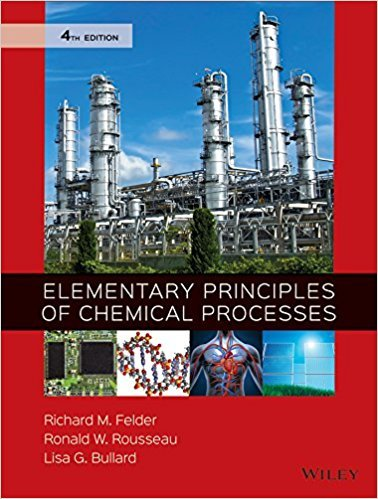×
×

# Solutions for Chapter 5.3a: EQUATIONS OF STATE FOR NONIDEAL GASES## Full solutions for Elementary Principles of Chemical Processes | 4th Edition

ISBN: 9780470616291Solutions for Chapter 5.3a: EQUATIONS OF STATE FOR NONIDEAL GASES

Solutions for Chapter 5.3a
4 5 0 373 Reviews
15
4
##### ISBN: 9780470616291

This expansive textbook survival guide covers the following chapters and their solutions. Since 3 problems in chapter 5.3a: EQUATIONS OF STATE FOR NONIDEAL GASES have been answered, more than 38111 students have viewed full step-by-step solutions from this chapter. Elementary Principles of Chemical Processes was written by and is associated to the ISBN: 9780470616291. This textbook survival guide was created for the textbook: Elementary Principles of Chemical Processes, edition: 4. Chapter 5.3a: EQUATIONS OF STATE FOR NONIDEAL GASES includes 3 full step-by-step solutions.

Key Chemistry Terms and definitions covered in this textbook
• actinide element

Element in which the 5f orbitals are only partially occupied. (Section 6.8)

Attraction between unlike molecules. (11.3)

• alkanes

Compounds of carbon and hydrogen containing only carbon–carbon single bonds. (Sections 2.9 and 24.2)

• angle strain

The increase in energy associated with a bond angle that has deviated from the preferred angle of 109.5°.

• complex lipid

A lipid that readily undergoes hydrolysis in aqueous acid or base to produce smaller fragments.

• compound.

A substance composed of atoms of two or more elements chemically united in fixed proportions. (1.4)

• constructive interference

When two waves interact with each other in a way that produces a wave with a larger amplitude.

• Diastereomers

Stereoisomers that are not mirror images of each other; refers to relationships among two or more objects

• electron domain

In the VSEPR model, a region about a central atom in which an electron pair is concentrated. (Section 9.2)

• exothermic

Any process with a negative DH (the system gives energy to the surroundings).

• Henderson–Hasselbalch equation

The relationship among the pH, pKa, and the concentrations of acid and conjugate base in an aqueous solution: pH = pKa + log 3base4 3acid4. (Section 17.2)

• kinetic energy

The energy that an object possesses by virtue of its motion. (Section 5.1)

• nitrogen rule

In mass spectrometry, an odd molecular weight indicates an odd number of nitrogen atoms in the compound, while an even molecular weight indicates either an even number of nitrogen atoms or the absence of nitrogen.

• nucleotide

Compounds formed from a molecule of phosphoric acid, a sugar molecule, and an organic nitrogen base. Nucleotides form linear polymers called DNA and RNA, which are involved in protein synthesis and cell reproduction. (Section 24.10)

• Order of precedence of functions

A ranking of functional groups in order of priority for the purposes of IUPAC nomenclature.

• oxidation–reduction (redox) reaction

A chemical reaction in which the oxidation states of certain atoms change. (Section 4.4; Chapter 20: Introduction)

• ribonucleic acid (RNA)

A polynucleotide in which ribose is the sugar component. (Section 24.10)

• sigmatropic rearrangements

A pericyclic reaction in which one s bond is formed at the expense of another.

• sulfonation

An electrophilic aromatic substitution reaction in which an SO3H group is installed on an aromatic ring.

• waxes

High-molecular-weight esters that are constructed from carboxylic acids and alcohols.

×